World Statistics Day was yesterday, but we’re celebrating all week long! This celebration means acknowledging the impact statistics has on our world. Who is your favorite statistician? Share with us who they are and why they top your favorites list.
Choose Language Hide Translation Bar
Empirical power calculations for designed experiments with 1-click simulate in JMP 13

When designing an experiment, a common diagnostic is the statistical power of effects. Bradley Jones has written a number of blog posts on this very topic. In essence, what is the probability that we can detect non-negligible effects given a specified model? Of course, there are a set of assumptions/specifications needed in order to do this, such as effect sizes, error, and significance level of tests. I encourage you to read some of those previous blog posts if you’re unfamiliar with the topic.

If our response is continuous, and we are assuming a linear regression model, we can use results from the Power Analysis outline under Design Evaluation. However, what if our response is based on pass/fail data, where we are planning to do 10 trials at each experimental run? For this response, we can fit a logistic regression model, but we cannot use the results in the Design Evaluation outline. Nevertheless, we’re still interested in the power...

What to do?
We could do a literature review to see about estimating the power, and hope to find something that applies (and do so for each specific case that comes up in the future). But, it is more straight-forward to run a Monte Carlo simulation. To do so, we need to be able to generate responses according to a specified logistic regression model. For each of these generated responses, fit the model and, for each effect, check if the p-value falls below a certain threshold (say 0.05). This has been possible in previous versions of JMP using JSL, but requires a certain level of comfort with scripting and in particular scripting formulas and extracting information from JMP reports. Also, you need to find the time to write the script. In JMP Pro 13, you can now perform Monte Carlo simulations with just a few mouse-clicks.

That sounds awesome
The first time I saw the new one-click simulate, I was ecstatic, thinking of the possible uses with designed experiments. A key element needed to use the one-click simulate feature is a column containing a formula with a random component. If you read my previous blog post on the revamped Simulate Responses in DOE, then you know we already have a way to generate such a formula without having to write it ourselves.

1. Create the Design, and then Make Table with Simulate Responses checked
In this example, we have four factors (A-D), and plan an eight-run experiment. I’ll assume that you’re comfortable using the Custom Designer, but if not, you can read about the Custom Design platform here. This example can essentially be set up the same way as an example in our documentation.

Before you click the Make Table button, you need to make sure that Simulate Responses has been selected from the hotspot at the top of the Custom Design platform.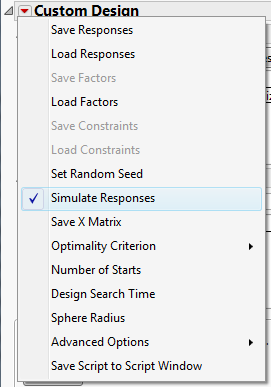2. Set up the simulation
Once the data table is created, we now have to setup our simulation via the Simulate Response dialog described previously. Under Distribution, we select Binomial, with and set N to 10 (i.e. 10 trials for each row of the design). Here, I’ve chosen a variety of coefficients for A-D, with factor D having a coefficient of 0 (i.e., that factor is inactive). The Simulate Response dialog I will use is: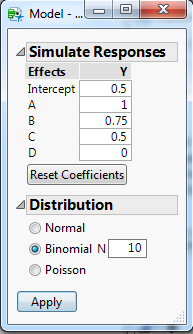Clicking the Apply button, we get a Y Simulated column simulating the number of successes out of 10 trials, and a column indicating the number of trials (which is used in Fit Model). For modeling purposes, I copied the Y Simulated column into Y.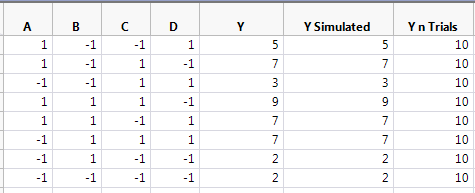If we look at the formula for Y Simulated, we see that it can generate a response vector based on the model given in the Simulate Responses dialog.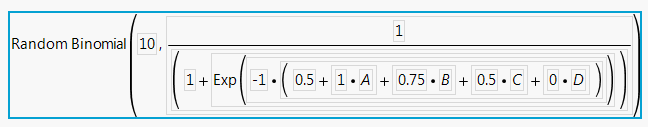3. Fit the Model
Now that we have a formula for simulating responses, we need to set up the modeling for the simulated responses. In this case, we want to collect p-values for the effects from repeated logistic regression analyses on the simulated responses. We first need to do the analysis for a single response. If we launch the Fit Model platform, we can add the number of trials to the response role (Y), and change the Personality to Generalized Linear Model with a Binomial Distribution. My Fit Model launch looks like this: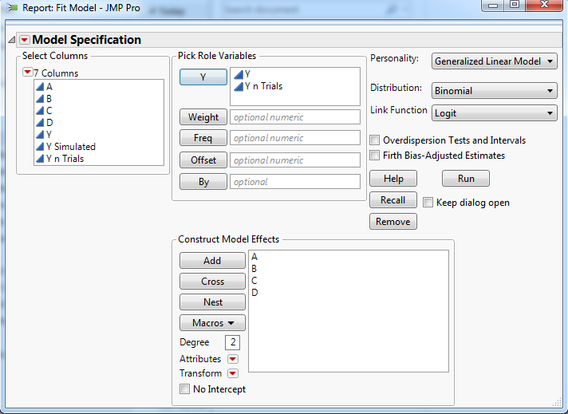Click the Run button to fit the model. The section of the report that we’re interested in is the Parameter Estimates outline.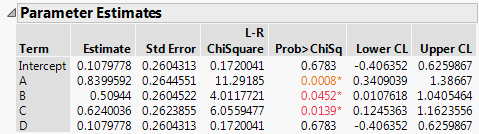For the initial simulated response, A, B, and C were found to be active, and D was not (which is correct). Of course, this is just for a single simulation. We could keep simulating a new response vector, and keeping track of these p-values for each effect, or, we could use one-click simulate and let it do this for us.

4. Run the simulation
The column we’re interested in for this blog post is the Prob>ChiSq. We right-click on that column to bring up the menu, and (if you have JMP Pro), at the bottom, above bootstrap, we see an option for Simulate.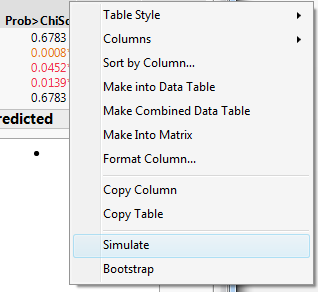The dialog that pops up has a choice for Column to Switch Out and a Choice for Column to Switch In. For our simulations, instead of using the response Y, we want to use Y Simulated, as it contains the formula with the Random Binomial. Instead of using Y when we first used Fit Model, we could have instead used Y Simulated, and switch it out with itself. The Number of Samples refers to how many times to simulate a response. Here I’ve left it at 2500.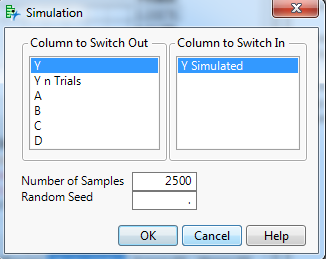Now we just click OK, and let it run. After a short wait, we’re presented with a data table containing a column for each effect from the Fit Model dialog (as well as a simulation ID, SimID), and 2501 rows – the first is the original fit, and marked as excluded, while each other row corresponds to the results from one of our 2500 simulated responses. The values are the p-values for each effect from Fit Model.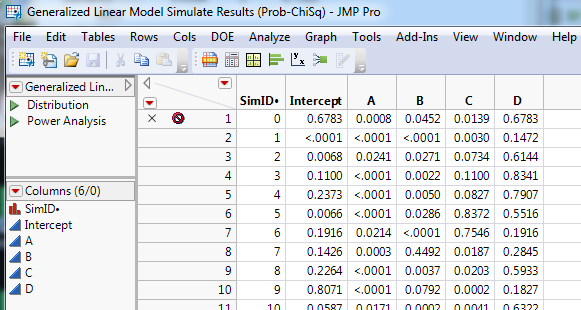The one-click Simulate has also pre-populated the data table with a distribution script, and, because it recognizes the results are p-values, another script called Power Analysis. Running the Power Analysis script provides a distribution of the p-values for each effect, as well as a summary titled Simulated Power with the rejection rate at different levels of alpha. For example, if we look at the effect of factor B, we see that at alpha = 0.05, 2103 times out of 2500 the null hypothesis of no effect was rejected for a rejection rate (empirical power) of about 84%.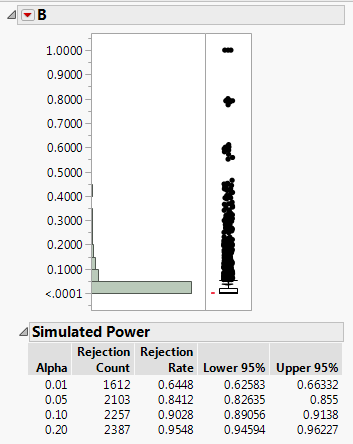I typically right-click on one of the Simulated Power tables, and select Make Combined Data Table. This provides a data table that provides the rejection rates for each term at the four different alpha levels. This makes it easier to view the results in Graph Builder, such as the results for alpha = 0.05.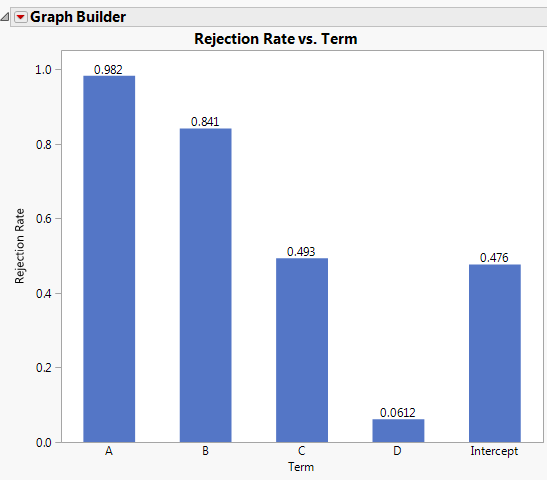Now we can see that we have high power to detect the effects for A and B (recall that they had the largest coefficients), while C and the intercept are around 50%. Since D was inactive in our simulation, the rejection rate is around 0.05, as we would expect. We may be concerned with 50% power assuming that the effect of C is correct. With the ease of being able to perform these simulations, it’s simple to go back to Simulate Reponses and change the number of trials for each row of the design before running another one-click simulation. Likewise, we could create a larger design to see how that affects the power. We could even try modeling using generalized regression with a binomial distribution.

Final thoughts
To me, a key aspect of this new feature is that it allows you to go through different “what if” scenarios with ease. This is especially true if you are in a screening situation, where it’s not unusual to be using model selection techniques when analyzing data. Now you can have empirical power calculations that match the analysis you plan to use, and help alert you to pitfalls that can arise during analysis. While this was possible prior to JMP 13, I typically didn’t find the time to create a custom formula each time I was considering a design. In the short time I’ve been using the one-click simulate, the ease with which I can create the formula and run the simulations has led me to insights I would not have gleaned otherwise, and has become an important tool in my toolbox.

Article Labels

There are no labels assigned to this post.

Article Tags